Saturday, October 23, 2021
HomeTest EquipmentsDigital VoltmeterVoltmeter - Working Principle, Voltage Sensitivity, Types and Applications

# Voltmeter – Working Principle, Voltage Sensitivity, Types and Applications

Voltmeter, since its invention, has always been a backbone of measurements of power circuitry. To ensure that your circuitry has been designed and assembled correctly, you must need voltage meter based measuring instruments to verify it. This post will discuss what is Voltmeter, its working principle, voltage equation, voltage sensitivity, various types of voltmeters and their applications.

## What is Voltmeter

A Voltmeter is a measuring instrument which measures the voltage between the two nodes in an electric circuit. In analog voltmeters the pointer moves across a scale in proportional to the voltage of the circuit. Digital voltmeters have a numerical display of voltage by using an analog to digital converter.

Permanently mounted voltmeters are used to monitor generators or other fixed apparatus. Portable instruments are equipped with multimeter to measure current and resistance. They are the standard test instruments used in electrical and electronics work.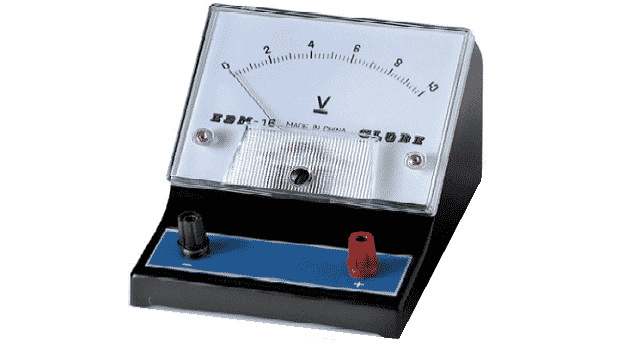Fig. 1 – Voltmeter

## Working Principle of Voltmeter

Its Working is based on the principle of Ohm’s law. Ohm’s law states that – “Voltage across a resistance is directly proportional to the current passing through it”. Any basic meter has a potential difference across its terminals when a full-scale current flows through it. The symbol to represent the voltmeter is a circle with an enclosed letter V.Fig. 2 – Circuit Diagram to Represent Voltage

A voltmeter is always connected in parallel to the component in a circuit for which the voltage is to be measured. A DC volt-meter has polarity signs on it. Therefore one has to connect the plus (+) terminal of the volt-meter to the higher point of potential and the minus (-) terminal to the lower point of potential in order to obtain a meter deflection.

In an AC volt-meter there is no polarity signs on it and can be connected anyways. However, in this case also, the voltmeter is still connected in parallel to the component for which the voltage is measured. A volt-meter with a high voltage range is built by connecting a resistance in series with a meter mechanism that has a full scale voltage capability as shown in the below Figure.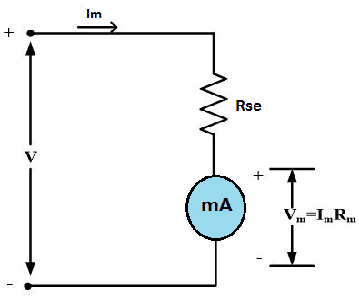Fig. 3 – Full Scale Voltage Capability

### Voltage Equation

The resistance in series is called a multiplier. Its value is determined from the Voltage Equation.

• V = Voltage,
• Im = Full scale current,
• Rse = Resistance in Series, and
• Vm = Full Scale Voltage

### Voltage Sensitivity

Voltage Sensitivity is the reciprocal of the current necessary for full scale deflection.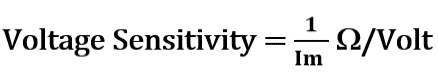The smaller the meter current, the larger the voltage sensitivity will be. The actual voltmeter resistance equals the sensitivity times the complete voltage. The voltmeter resistance will always be constant even though the voltage reading may not be a full-scale reading.

## Types of VoltmetersFig. 4 – Types of Voltmeters

### 1. Analog Voltmeter

It includes deflecting type of indicating voltage meters. An Analog Voltmeter can be categorized in three categories. They are:

• Moving Coil Instruments
• Moving Iron Instruments
• Electrostatic VoltmeterFig. 5 – A functional View of Analog Voltmeter

#### 1.1. Moving Coil Instruments

Moving Coil Instruments type Analog Voltmeters are available in two types. They are:

• Permanent Magnet Type Moving Coil Instruments
• Dynamo Meter Type Moving Coil Instruments

#### 1.1.1. Permanent Magnet Type Moving Coil Instruments

Permanent magnet type Moving Coil Instruments respond only to direct current. These instruments have permanent magnet to produce magnetic field. The coil is wounded on a soft iron piece and it rotates in its own vertical axis. When the current flows through the coil, deflecting torque is generated according to Lorenz force equation.Fig. 6 – Moving Coil Voltmeter

#### 1.1.2. Dynamo Meter Type Moving Coil Instruments

Dynamo meter type Moving Coil Instruments consist of two coils. One coil is fixed and the other coil rotates around it. The interaction of the two fields produces a deflecting torque.

#### 1.2. Moving Iron Instruments

Moving Iron Instruments are used in AC circuits and are categorized into Simple Moving Iron, Dynamo Meter type and Induction types of instruments. It consists of soft iron containing movable and fixed coils.Fig. 7 – Moving Iron Voltmeter

The interaction of fluxes produced by these elements produces deflecting torque. The ranges are extended by keeping the resistors in series with the coil.

#### 1.3. Electrostatic Voltmeter

It works on the electrostatic principle where the repulsion between two charges plates deflect at a pointer attached to a spring.

These instruments are used for high voltage AC and DC measurements. These are high sensitive instruments and are capable of measuring smallest charge voltages as well as high range voltage nearly 200KV.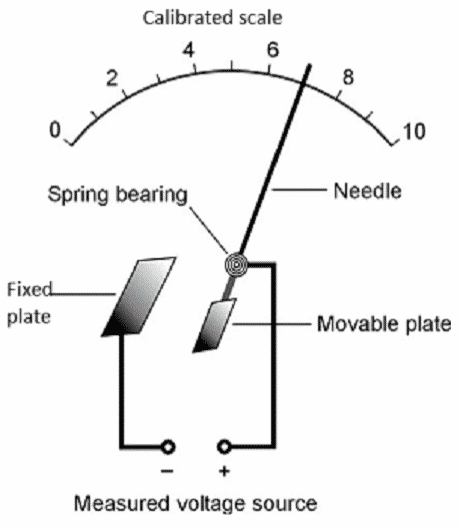Fig. 8 – Electrostatic Voltmeters

### 2. VTVMs and FET VMs

These types of instruments can handle both AC/ DC voltages and resistance measurements. These devices use electronic amplifier in between the input and the meter.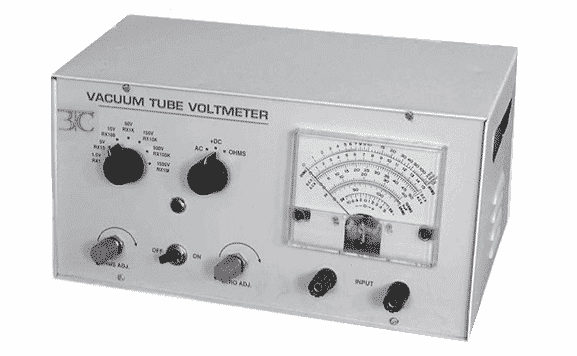Fig. 9 – Vacuum Tube Voltmeter

If this device uses the vacuum tube in the amplifier then it is called as Vacuum Tube Voltmeter (VTVM). VTVM’s are used in high power AC measurements.

Field Effect Transistor (FET) is a transistor that uses electric field to control the electrical behavior of the device. They are also known as unipolar transistors. Field Effect Transistor based Voltmeter utilizes this property of field effect transistors while measuring voltage.

### 3. Digital Voltmeter (DVM)

DVM displays the voltage using LEDs or LCDs to display the result. The instrument has to contain an A/D converter. The device contains programmed micro controller, ADC and LCD display, to provide accurate digital display of analog values from 0 to 15 volts DC.Fig. 10 – Digital Voltmeter and Multimeter

They are used because of the properties like accuracy, durability and reduces parallax errors.

`Read more about Digital Voltmeter, its principle and how it works in detail.`

## Applications of Voltmeter

The applications of Voltmeter include:

• It is very useful in finding the voltage of a charge storing device, such as to test the voltage of a battery. For example, a new AAA cell will have about 1.6V, a dying one perhaps 1.1V. A 12V lead-acid car battery will read 12.5V when fully charged, or 14V when being charged by the alternator in a car. If it reads 10V, there is something wrong with the alternator.
• It can be used just to tell whether there is power in a circuit or not, such as a mains outlet.
• Verifying the power is on or off to the appliances.
• We can calculate the current by measuring the voltage across a known resistance. This is useful when you don’t have an ammeter.
• They are used to build a continuity checker, with a series battery.
• They are used to build an ohm meter, by using a voltage divider with the unknown resistor.
• They are used to build an ammeter by measuring the voltage across a shunt resistor.
```Also Read:
What is Digital Voltmeter - How it Works, Types, Applications, Advantages
Power Factor - Power Triangle, Types, PFC, Applications, Advantages
RELATED ARTICLES